# Grade - math word problems

#### Number of problems found: 5482

• Collection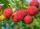John has collected half a bucket of raspberries in 45 minutes, Eva collects the whole bucket in an hour. How many minutes it would take to fill a bucket if the two children work together?
• Chord distanceThe circle k (S, 6 cm), calculate the chord distance from the center circle S when the length of the chord is t = 10 cm.
• Water containerContainer with water weighs 1.48 kg. When we cast 75% of water container of water weight 0.73 kg. How heavy is an empty container?
• EricaErica bought 3 1/2 yards of fabric. If she uses 2/3 of the fabric, how much will she have left?
• Circle - AGFind the coordinates of circle and its diameter if its equation is: ?
• Runner 2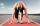Marian run 12 meters in 8 seconds. How far would Marian run for 70 seconds, if still run at the same pace?
• Mr and MrsMr. Calda and Mrs. Cald have a total of 139 years. How many years have when we know that Mr. Calda is 9 years older than Mrs. Cald
• Train from BrnoFirst train started from Brno at 8 am at 60 km per hour. Second train started from Opava at 7 o'clock at the speed of 40 kilometers per hour. In how many hours and how far from cities trains meet when the distance of cities is 200 kilometers.
• Christmas trees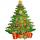Salesman sold Christmas trees: spruce for € 22, pine for € 25 and fir for € 33. At the morning he had the same number of spruce, fir and pine. At the evening he had all the trees entirely sold for € 3,600. How many trees that day salesman sold?
• ContainerThe container-shaped box with internal dimensions of 3.9 m, 3.25 m and 2.6 m was completely filled with goods in the same cubic boxes. How long edge could this box have?
• Percentages 5\$5.25 is 7 1/2% of what number?
• Cuboid 5Calculate the mass of the cuboid with dimensions of 12 cm; 0.8 dm and 100 mm made from spruce wood (density = 550 kg/m3).
• Internal anglesOne internal angle of the triangle JAR is 25 degrees. The difference is the size of the two other is 15°. Identify the size of these angles.
• GP - three membersThe second and third of a geometric progression are 24 and 12(c+1) respectively, given that the sum of the first three terms of progression is 76 determine value of c
• Pupils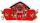Two classes to collect money. Boys are four sevenths pupils. In time did not pay a quarter of the boys and a sixth of girls, which together mean 12 sinners. How many pupils attend this two classes ?
• 3sides prismThe base of vertical prism is an isosceles triangle whose base is 10 cm and the arm is 13 cm long. Prism height is three times the height of base triangle. Calculate the surface area of the prism.
• Proportion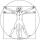write the following proportion 8 is to 64 as to 2 is to x
• Water pool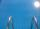Pool with volume 990hl completely filled, if water flows by one tap 8 hours and by second tap 6 hours. First tap give 10hl more than second per hour. How many hl flows in each of them in an hour?
• Cuboid - VabFind the surface of the cuboid when its volume is 52.8 cubic centimeters, and the length of its two edges is 2 centimeters and 6 centimeters.
• Percentage and rectangleAbout what percentage increases perimeter and area of a rectangle if both the sides 12 cm and 10 cm long we increase by 20%?

Do you have an interesting mathematical word problem that you can't solve it? Submit a math problem, and we can try to solve it.

We will send a solution to your e-mail address. Solved examples are also published here. Please enter the e-mail correctly and check whether you don't have a full mailbox.

Please do not submit problems from current active competitions such as Mathematical Olympiad, correspondence seminars etc...